In :
%matplotlib inline
import matplotlib.pyplot as plt
import seaborn as sns
plt.rcParams['axes.grid'] = False

import logging
logger = logging.getLogger()


# 20 van Emde Boas Trees¶

data structures that support the operation of a prioprity queue:

1. base its decisions on comparing keys, sorting bound $\Omega(n \lg n)$ $\to$ at least one important opeartion took $O(\lg n)$ time.

2. exploit additional information (like counting sort): $k \in [0,1,\dotsc,n-1]$, with no duplicates allowed. $\to$ van Emde Boas trees $O(\lg \lg n)$.

To avoid any further confusion, define:
$n$ - the number of elements currently in the set;
$u$ - universe size, namely, the size of universe $\{0,1,2,\dotsc,u-1\}$.

We assumen throughout that $u$ is an exact power of 2, i.e., $u = 2^k$.

### 20.1 Preliminary approaches¶

examine various approaches for sorting a dynamic set.

We maintain an array $A[0\dotso u-1]$. The entry $A[x]$ holds a 1 if the value $x$ is in the dynamic set, and it holds a 0 otherwise.

INSERT, DELETE, MEMBER: $O(1)$
MINIMUM, MAXIMUM, SUCCESSOR, PREDECESSOR: $O(u)$

#### Superimposing a binary tree structure¶

We can short-cut long scans in the bit vector by superimposing a binary tree of bits on top of it. The bit stored in an internal node is the logical-or of its two children.

In :
plt.imshow(plt.imread('./res/fig20_1.png'))

Out:
<matplotlib.image.AxesImage at 0x1096ebcf8>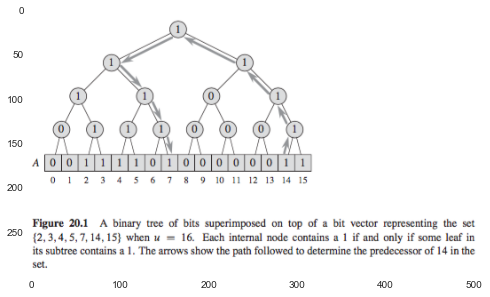INSERT, DELETE, MEMBER: $O(1)$
MINIMUM, MAXIMUM, SUCCESSOR, PREDECESSOR: $O(\lg u)$

This approach is only marginally better than just using a red-black tree.
The MEMBER is $O(1)$, whereas a red-black needs $O(\lg n)$.
If $n$ is much smaller than $u$, a red-black tree would be faster for all the other operations.

#### Superimposing a tree of constant height¶

We superimpose a tree of degree $\sqrt{u}$, the height of the resulting tree is always 2.

Each internal node stores the logical-or of the bits within its subtree

In :
plt.imshow(plt.imread('./res/fig20_2.png'))

Out:
<matplotlib.image.AxesImage at 0x10a131da0>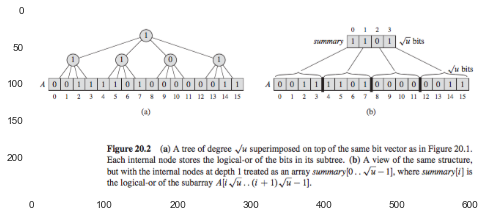INSERT, DELETE, MEMBER: $O(1)$
MINIMUM, MAXIMUM, SUCCESSOR, PREDECESSOR: $O(\sqrt{u})$

Using a tree of degree $\sqrt{u}$ will turn out to be a key idea of van Emde Boas trees.

In :
# Exercises


### 20.2 A recursive structure¶

idea: $T(u) = T(\sqrt{u}) + O(1) \to T(u) = O(\lg \lg u)$

We assume $u = 2^{2^k}$, and make the structure in Fig.20.2 recursive, shrinking the universize size by the square root at each level of recursion.

define:
\begin{align} high(x) &= \lfloor x / \sqrt{u} \rfloor \\ low(x) &\equiv x \mod \sqrt{u} \\ index(h, l) &= h \sqrt{u} + l \\ \end{align}

#### 20.2.1 Proto van Emde Boas structures¶

• if $u = 2$, then it is the base size, and it contains an array $A[0\dotso1]$ of two bits.

• Otherwise, $u = 2^{2^k}$. Its structure is illustrated in Fig.20.3:

In :
plt.imshow(plt.imread('./res/fig20_3.png'))

Out:
<matplotlib.image.AxesImage at 0x108d84080>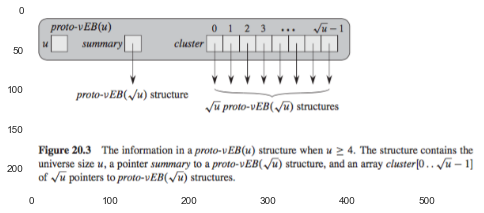In :
plt.figure(figsize=(8,12))

Out:
<matplotlib.image.AxesImage at 0x10a3720f0>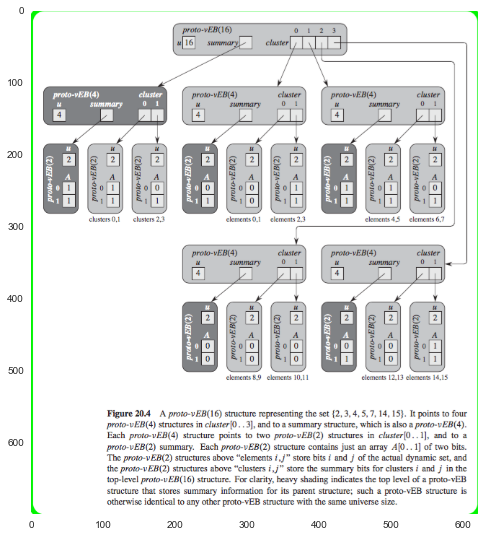#### 20.2.2 Operations on a proto van Embe Boas structure¶

##### Determining whether a value is in the set¶

$O(\lg \lg u)$

In :
plt.imshow(plt.imread('./res/member.png'))

Out:
<matplotlib.image.AxesImage at 0x10b36a780>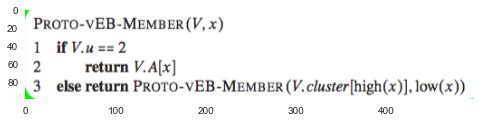##### Finding the minimum element¶

$O(\lg u)$

In :
plt.imshow(plt.imread('./res/find_min.png'))

Out:
<matplotlib.image.AxesImage at 0x10ba1a400>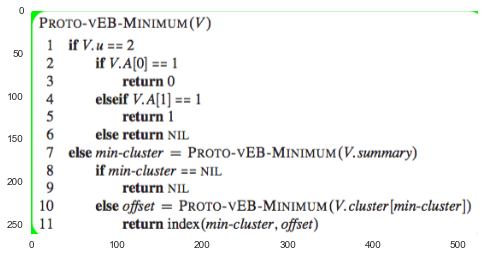##### Finding the successor¶

$O(\lg u \lg \lg u)$

In :
plt.imshow(plt.imread('./res/find_successor.png'))

Out:
<matplotlib.image.AxesImage at 0x10cac2390>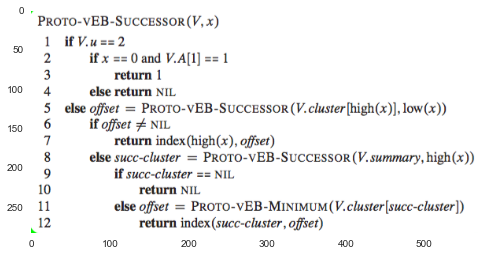##### Inserting an element¶

$O(\lg u)$

In :
plt.imshow(plt.imread('./res/insert.png'))

Out:
<matplotlib.image.AxesImage at 0x10d27de10>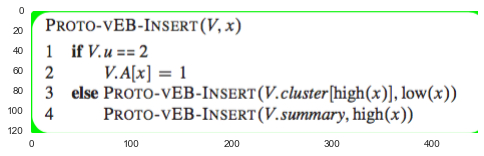##### Deleting an element¶
In :
#Exercises


### 20.3 The van Emde Boas tree¶

The proto-vEB structure of the previous section falls short because we have to recurse too many times in most of the operations.
$\to$ stores a little more information to removing the need for some of the recursion.

We will allow the universe size $u$ to be any exact power of 2, and when $\sqrt{u}$ is not an integer, we will divide the $\lg u$ bits into $\lceil (\lg u) / 2 \rceil$ and $\lfloor (\lg u) / 2 \rfloor$.

In :
plt.imshow(plt.imread('./res/func.png'))

Out:
<matplotlib.image.AxesImage at 0x109623dd8>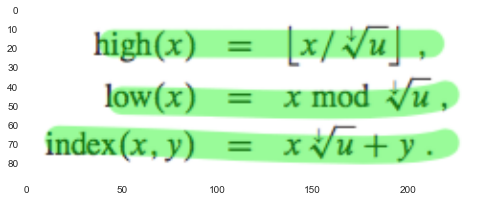#### 20.3.1 van Emde Boas trees¶

a vEB tree contains two attributes not found in a proto-vEB structure:

• min: stores the minimum element in the vEB tree.
the element stored in min does not appear in any of the recursive vEB trees that the cluster array points to.

• max: stores the maximum element in the vEB tree.

These attributes will help us in four ways:

1. The MINIMUM and MAXIMUM operations do not even need to recurse.

2. The SUCCESSOR operation can avoid making a recursive call to determine whether the successor of a value $x$ lies within high(x).

3. We can tell whether a vEB tree has no elements, exactly one element, or at least two elements in constant time from its min and max values.

4. INSERT and DELETE can be operated in constant time if the number of its elements is known.

In :
plt.figure(figsize=(10,15))

Out:
<matplotlib.image.AxesImage at 0x10ac0d588>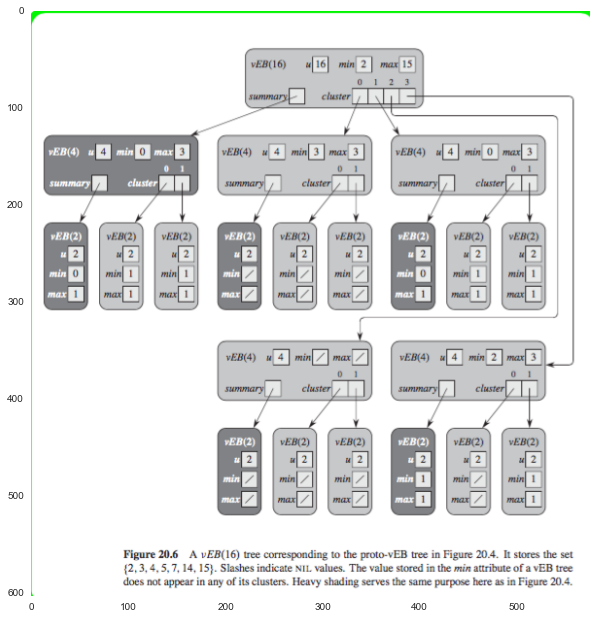#### 20.3.2 Operations on a van Emde Boas tree¶

##### Finding the minimum and maximum elements¶

$O(1)$

In :
plt.imshow(plt.imread('./res/veb_find_min.png'))

Out:
<matplotlib.image.AxesImage at 0x10bb546d8>